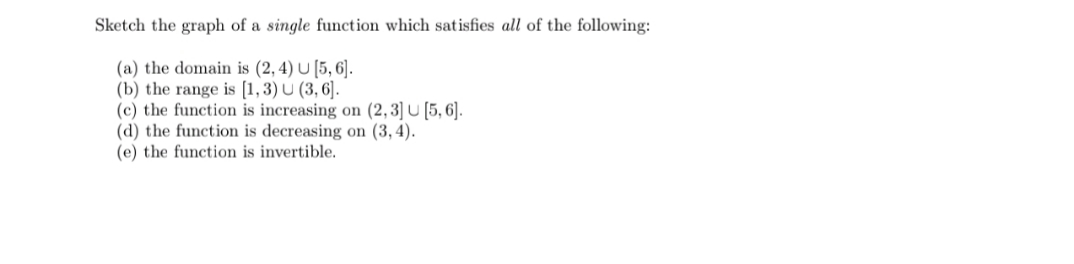# Sketch the graph of a single function which satisfies all of the following: (a) the domain is (2,4) U [5, 6] (b) the range is [1,3) U (3, 6]. (c) the function is increasing on (2, 3] U [5, 6]. (d) the function is decreasing on (3,4). (e) the function is invertible

Questionhelp_outlineImage TranscriptioncloseSketch the graph of a single function which satisfies all of the following: (a) the domain is (2,4) U [5, 6] (b) the range is [1,3) U (3, 6]. (c) the function is increasing on (2, 3] U [5, 6]. (d) the function is decreasing on (3,4). (e) the function is invertible fullscreen

### Want to see the step-by-step answer?